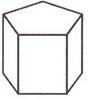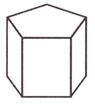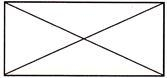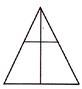Courses

Test: Shapes (Geometry) - 2

10 Questions MCQ Test Mathematics for Class 3: NCERT | Test: Shapes (Geometry) - 2

Description
Attempt Test: Shapes (Geometry) - 2 | 10 questions in 20 minutes | Mock test for Class 3 preparation | Free important questions MCQ to study Mathematics for Class 3: NCERT for Class 3 Exam | Download free PDF with solutions
QUESTION: 1

Directions: Consider the figure shown below:The number of edges in the figure is ________

Solution:

Total number of edges in the given figure are 15

QUESTION: 2

Solution:
QUESTION: 3

Directions: Consider the figure shown below:The number of vertices in the figure is

Solution:
QUESTION: 4

If two lines never meet anywhere and distance between the lines is always equal, then the lines are called:

Solution:
QUESTION: 5

Identify the line segment from the given options.

Solution:
QUESTION: 6

Consider the following statements:
Statement 1: A line segment has fixed length and it cannot be extended.
Statement 2: A ray has fixed length and it cannot be extended. Which one of the following is correct about the above statements?

Solution:
QUESTION: 7

A line is produced to meet another line at a point O. Which one of the following is correct about the point O.

Solution:
QUESTION: 8

An arrow mark at the end of the line represents which one of the following?

Solution:
QUESTION: 9

How many triangles are there in the figure below?Solution:
QUESTION: 10

In the following figure, two lines are intersecting each other. Find the number of triangles formed in the given figure?Solution:Use Code STAYHOME200 and get INR 200 additional OFF Use Coupon Code

Track your progress, build streaks, highlight & save important lessons and more!

Similar ContentRelated tests